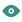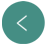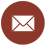• #### Archimedean screws and systems for raising water, from Leonardo da Vinci, Codex Atlanticus, f. 26 v. Milan, Biblioteca Ambrosiana.

Home | Didactics | Classes | Hydraulics (M.D. 509) - First Level Degree in Civil Engineering, Environmental Engineering and Industrial Engineering (Taranto)Didactics

Classes

# Hydraulics (M.D. 509) - First Level Degree in Civil Engineering, Environmental Engineering and Industrial Engineering (Taranto)1243Scientific Sector
ICAR/01 Hydraulics

Number of University Credits
6

Educational Goals
The course deals with fundamental issues in the Degree Class of Civil Engineering, Environmental Engineering and Industrial Engineering, of which is an essential and distinctive educational activity. The teaching of hydraulics provides engineering students with the basics of the fluid mechanics and tools of the applied hydraulics. The program is divided into three parts. In the first part the basic principles of fluid mechanics and hydraulics laws governing the fluid motion are provided. In the second part the typical applications of steady flows in pipes, local and net head loss and the unsteady flows in are presented. In the third part the course provides the students with the basics of the steady flows in open channels. In addition, students are given the skills necessary for understanding the fluid dynamics through laboratory training.

Summary of the program
BASICS OF HYDRAULICS (2.5 credits)
• Preliminary remarks. Physical properties of fluids. Hydrostatic.
• Equilibrium of a fluid element and integral relation for a control volume.
• Hydrostatic forces on plane and curved surfaces.
• Kinematics of fluids. Conservation of mass with differential and integral relations.
• Dynamics of frictionless and Newtonian flows. Turbulent flows. Differential and integral equations of linear momentum. Forces in dynamic conditions.
• VISCOUS FLOW IN DUCTS (2.5 credits)
• Velocity and discharge measurements.
• Steady flows in long circular ducts.
• Total and local head losses.
• Introduction of the unsteady flow in ducts.
• OPEN-CHANNEL FLOWS (1 credit)
• Steady flows in open channels.
• Specific energy.
• Uniform flows and design of channels.
Preparatory exams
Physics and Theoretical Mechanics.

Lab training requirements
The activities of lab training are required, since they are fundamental also the theoretical part of the course.

Structure in different typological educational activities

 Educational activities Credits Didactics (hours) Studying (hours) Theoretical classes 4 32 68 Excecises 1 16 9 Laboratory training 0.5 12 0.5 Seminar 0.5 12 0.5 Total 6 72 78

Final examination
The final exam of Hydraulics is composed of:

1) Three written tests on each of the following subjects: a) hydrostatic forces, b) dynamic forces and c) design of multiple-pipe systems and channels.
2) Oral test on the theoretical part of the course.
3) Lab training (the lab experiments are carried out only during the course at the Hydraulic Laboratory of the faculty).

During the classes three written tests (exemptions from the examination written tests) will be proposed to the students who attend the lessons (always on each of the following subjects: a) hydrostatic forces, b) dynamic forces and c) Design of multiple-pipe systems and channels). The students that pass these three tests will be successively examined only orally on the theoretical  part of the course. In case of fail of one or more of the exemption tests (also for absence), the students will be examined also with the relating written tests.
During the final examination the students will present also a report describing the laboratory experiments on the calibration of a Venturi meter and a weir.

Detailed program of the course
Basics of fluids. Difference of characteristics between solids, liquids and gases. Shear stresses in fluids. Proof of the theorem of Cauchy tetrahedron. Physical properties of fluids: density and specific gravity.
Corollary of the theorem of Cauchy tetrahedron; hydrostatic case: the principle of Pascal. Compressibility of liquids. Compressibility of gas. Index number of Mach. Introduction to surface tension: definition; practical cases. Contact angle effects. Liquids wetting and notwetting the solid. Proof of the equation of Laplace.
Proof of the law of Jurin-Borelli: cases of the liquids that wet and notwet the solid. Dynamic and kinematic viscosity. Demonstration of the Law of Netwon.
Non-Newtonian fluids. Fluids with time-independent behavior (Bingham, dilatant and pseudoplastic). Fluids with time-dependent behavior (thixotropic and rheopectic). Other fluids.
Static of fluids: differential equation of hydrostatics (with proof); case of the gravitational field.
Integral static relation for a control volume (with proof). Stevin's law (with proof). Gage pressure and absolute pressure. Negative gage pressure. Gage and absolute pressure distribution on a plane. Hydrostatic forces on plane surfaces. Proof of equation of the center of pressure and its difference with the center of gravity.
Proof of the principle of operation of a simple open manometer. Proof of the principle of operation of a differential manometer.
Hydrostatic forces on curved surfaces. Basics. Case of curved surfaces with curved contour line lying on a plane. Cases of concave or convex surfaces.
Fluids with low specific weight. Proof of the Mariotte formula.
Kinematics of fluids. Regimes of viscous flows: laminar, transitional and turbulent. Experiment of Reynolds. Reynolds number index. Eulerian and Lagrangian descriptions. Total derivative. Trajectories, streamlines and smokelines.
Types of flows: steady, unsteady, gradually varied, uniform. Concept of uniform flow extended to the turbulent motions. Proof of the differential equation of mass conservation for compressible fluids. Case of incompressible fluids.
Integral mass conservation equation for a control volume. Case of an incompressible fluid.
Fluid dynamics. Differential equation of linear momentum of frictionless flows (Euler equation).
Proof of the Bernoulli theorem of Bernoulli. Geometrical and energy meaning of the Bernoulli theorem.
Flows issuing from slots: cases of the slot at the tank bottom or at the vertical wall of the tank. Sluice gate. Rectangular weir (with proof).
Venturi meter and other Bernoulli-type devices. Pitot tube.
Integral equation of linear momentum of frictionless flows and its applications. Proof of the wall shear stress in ducts. Hydraulic radius.
Net applied force on fixed or movable jet-turning vane.
Extension of Bernoulli's theorem to the case of viscous fluids. Pipe head loss. Localized head loss. Energy grade line and hydraulic grade line: their geometrical and energy meaning.
Moody’s chart.
Darcy-Weisbach's law. Friction factor. The hydraulically smooth and rough walls: linear viscous sublayer. Friction factor for laminar flow, flows in the transition zone and in fully developed turbulent flows. Equation of Coolebrok-White. Darcy's law, formulae of Bazin, Kutter, Gauckler-Strickler and Manning.
Ducts with commercial diameters. Solution methods for piping systems in series and parallel. Open networks of pipe. Economy method of Marzolo.
Design of pipes with a pump. Economy formula of Bresse. Power of a pump. Pipe with uniform issuing of the discharge.
Unsteady flow in pipes. Phases of the water hammer in the case of abrupt pipe closure. Periodicity of the phenomenon in the case of frictionless flows. Overpressure of water hammer (with proof) and celerity of the water hammer (with proof).
Open-channel flows. Hydraulic grade line and energy grade line. Definition of specific energy. Uniform motion in the channels. Formula of Chézy. Open-channel design problems. Criterion of economy.
Exercises: Exercises on hydrostatic forces on plane and curved surfaces. Exercises on the Bernoulli's theorem and the dynamic forces. Exercises on the open pipe network design. Exercises on the open-channel flows.
Lab training: calibration of a Venturi meter and a weir.

Recommended book
M. Mossa, A.F. Petrillo, Idraulica, ISBN 978-88-08-18072-8, Casa Editrice Ambrosiana, Milano, 2013 (in Italian).

Other books
F.M. White, Fluid Mechanics, McGraw-Hill, 4th edition, 1999.
Y.A. Çengel, J.M. Cimbala, Mechanics of fluids, McGraw-Hill, 2011 (2nd edition).
D. Citrini, G. Noseda, Idraulica, Ed. CEA, Milano (in Italian).
A. Ghetti, Idraulica, Edizioni Libreria Cortina, Padova, 1981, 2nd edition (in Italian).
E. Marchi, A. Rubatta, Meccanica dei Fluidi: principi ed applicazioni idrauliche, UTET, Torino, 1981 (in Italian).
G. Alfonsi, E. Orsi, Problemi di idraulica e meccanica dei fluidi, Ed. CEA, Milano (in Italian).Prof.
Michele Mossa
PhD
Professor of Hydraulics at the
Polytechnic University of Bari
POLYTECHNIC UNIVERSITY OF BARI
DICATECh
Department of Civil, Environmental, Land, Building Engineering and Chemistry
Via E. Orabona, 4 - 70125 Bari - ITALY

www.dicatech.poliba.it

LIC
Coastal Engineering Laboratory
Area Universitaria di Valenzano